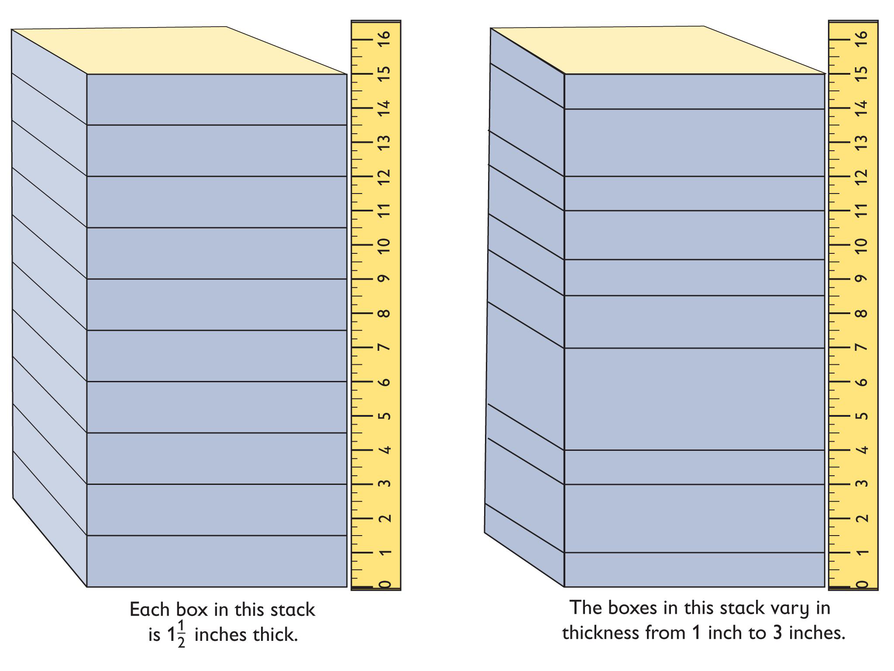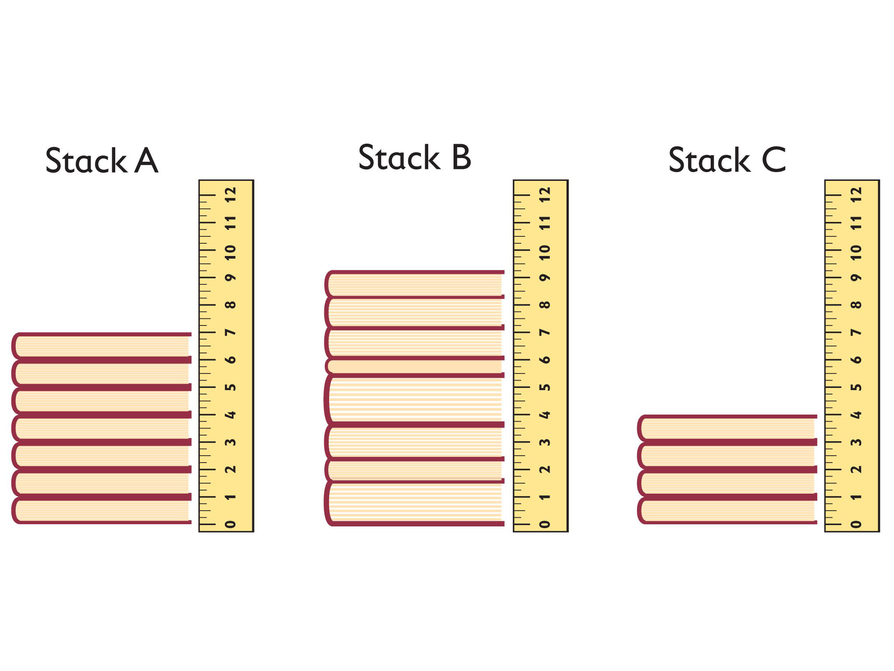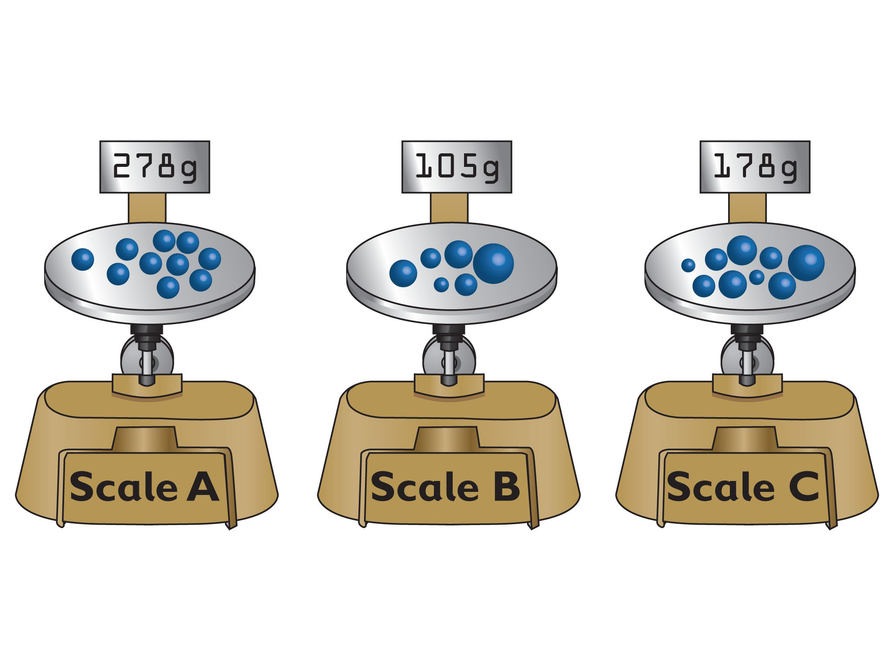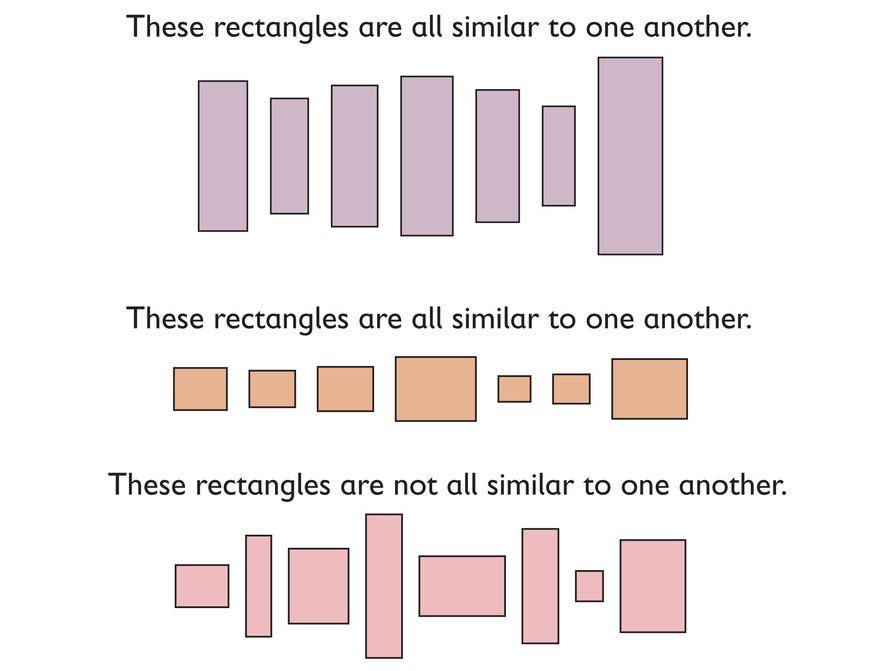Subject:
Numbers and Operations
Material Type:
Lesson Plan
Level:
Middle School
7
Provider:
Pearson
Tags:
7th Grade Mathematics, Estimation, Height, Measurement, Weight
Language:
English
Media Formats:
Text/HTML

# Exploring Numerical Relationships## Overview

Students are asked whether they can determine the number of books in a stack by measuring the height of the stack, or the number of marbles in a collection of marbles by weighing the collection.

Students are asked to identify for which situations they can determine the number of books in a stack of books by measuring the height of the stack or the number of marbles in a collection of marbles by weighing the collection.

# Key Concepts

As students examine different numerical relationships, they come to understand that they can find the number of books or the number of marbles in situations in which the books are all the same thickness and the marbles are all the same weight. This “constant” is equal to the value $\frac{B}{A}$ for a ratio A : B; students begin to develop an intuitive understanding of proportional relationships.

# Goals and Learning Objectives

• Explore numerical relationships

SWD: Some students with disabilities will benefit from a preview of the goals in each lesson. Have students highlight the critical features or concepts to help them pay close attention to salient information.

# Lesson Guide

• Introduce the situation about the stacks of boxes.
• Have students discuss with a partner the question, “For which stack of boxes can you determine the number of boxes in the stack by measuring the height of the stack?”
• Have students share ideas about the strategies and challenges in finding the number of boxes in the stacks.

# Teacher Demonstration

Demonstrate with an actual stack of boxes or books, either with equal or unequal thicknesses, or one stack of each.

# Mathematics

The significance of the fact that the boxes in the first stack have equal thicknesses is the central mathematical idea of the situation. It means that the same number of inches—in this case 112 inches—is a constant value that can be multiplied a certain number of times to reach a certain height. With boxes of unequal thicknesses, there is no constant value to multiply to reach a certain height. Ask your students the following:

• If the boxes in the first stack were each 1 inch thick, what would the height of the stack tell you?
• What if the boxes in the first stack were each 3 inches thick?
• Why would you ever need to figure out the number of things in a stack without counting them?
• Suppose there is a stack of more than 1,000 boxes of equal thicknesses. Could you figure out exactly how many boxes there are—without counting them—if you knew the height of the stack?
• Suppose there is a stack of more than 1,000 boxes with unequal thicknesses. Is there any way to figure out exactly how many boxes there are—without counting them—if you knew the height of the stack?

SWD: Students with disabilities may not as readily connect new information to previously learned skills and concept. Activate background knowledge about multiplying and dividing fractions to promote connections between students’ knowledge and the new topic being investigated here.

# Stacks of Boxes

Discuss the following with your classmates.

• For which stack of boxes can you determine the number of boxes in the stack by measuring the height of the stack?# Lesson Guide

Discuss the Math Mission. Students will identify groups of objects for which the number of objects in the group can be determined by using numerical relationships instead of counting.

## Opening

Determine the number of objects in a group by using numerical relationships instead of counting.

# Lesson Guide

Have students work in pairs.

SWD: Students with disabilities may struggle to self-monitor whether strategies are effective or ineffective. If students are demonstrating difficulty shifting gears from an ineffective strategy, model Think-Aloud strategies to help students to reflect.

# Mathematical Practices

Mathematical Practice 7: Look for and make use of structure.

Look for students who are relying heavily on the pictures to make sense of the situation and who are looking for structure within the visual representations for clues about the structure of the mathematics.

Mathematical Practice 2: Reason abstractly and quantitatively.

Identify students who are moving in and out between the concrete cases illustrated in each problem and generalizations about the relationship between the unit height (weight) and the total height (weight).

# Interventions

Student has difficulty getting started.

• What can you tell from the picture, and what are you trying to figure out?

Student doesn’t know what to focus on in each situation.

• Refer back to the stacks of boxes at the start of the lesson. What did you need to know to find out how many boxes were in a stack?
• Draw your own diagram of a stack of books, each with a thickness of 1 centimeter. What can you say about the height of the stack?
• Now draw your own diagram of a stack of books with unequal thicknesses. How does this compare with the height from before?

Student develops an approach and makes headway into the problems, but gets stuck and gives up.

• If you have a bunch of books that are each 1 centimeter thick, how tall is a stack of 10 books? 20 books? 100 books?

Student struggles to sort out the relevant operations—what is multiplied by what and what is divided by what.

• Congratulations! You are grappling with the important mathematics of the situation. Can you represent the various relationships you have identified among the quantities by using a set of equations?

• I could find the number of books in the stacks of books that have equal thicknesses, such as those in Stack A and Stack C.
• I would also need to know the thickness of a single book.

# Stacks of Books

• For which stack(s) of books could you determine the number of books in the stack by measuring the height of the stack?
• For the stack(s) of books you identified, what other information do you need to know to determine the number of books in the stack?## Hint:

• What would have to be true about the books in the stack for you to be able to tell how many books are in the stack?
• Think about what you would divide the height of the books by to find the number of books in the stack.

# Preparing for Ways of Thinking

Listen and look for students who:

• Talk about something being “constant” in each situation.
• Sort out what is being multiplied by what and what must be divided by what.

# Interventions

Student has difficulty getting started.

• What can you tell from the picture, and what are you trying to figure out?

Student doesn’t know what to focus on in each situation.

• Draw your own diagram of a collection of marbles that each weigh 1 ounce. What can you say about the weight of the collection?
• Now draw your own diagram of a collection of differently sized marbles. How does this compare with the weight from before?

Student develops an approach and makes headway into the problems, but gets stuck and gives up.

• If you have a bunch of marbles that each weigh 1 ounce, how much does a collection of 10 marbles weigh? 20 marbles? 100 marbles?

Student struggles to sort out the relevant operations—what is multiplied by what and what is divided by what.

• Congratulations! You are grappling with the important mathematics of the situation. Can you represent the various relationships you have identified among the quantities by using a set of equations?

• I could find the number of marbles in the collections of marbles that are of equal size (weight), such as those on Scale A.
• I would also need to know the weight of a single marble.

# Challenge Problem

• The first and second group of rectangles have a constant ratio because the rectangles are similar.
• Besides the ratio, you would need one of the sides of the rectangle to figure out the other side from the ratio. You would then multiply the two sides to find the area.

# Marbles

• For which collection(s) of marbles could you determine the number of marbles in the collection by measuring the weight of the collection?
• For the collection(s) of marbles you identified, the total weight of the marble collection is given. What other information would you need to determine the number of marbles in the collection if you couldn't count the marbles?# Challenge Problem

• For which group(s) of rectangles is the ratio of the length to the width constant?
• Given the ratio, what is the least amount of information you would need to know to find the area of one of the rectangles? Explain.## Hint:

What would you need to know about one marble to determine how many marbles there are?

# Mathematics

Have at least two pairs of students present their work for each of the problems. Draw out the explanations from their reasoning process as much as possible.

Make public (on the board, overhead, or through the tablets) several different answers to each of the following questions, either during or after student presentations.

• What is the difference between the stacks of books and collections of marbles whose height or weight determine the number of items, on the one hand, and the stacks and collections whose height or weight don’t tell you how many items there are in them?
• What operations are relevant in these problems? What is multiplied by what, and what can be divided by what?
• For the stacks of books and collections of marbles whose height and weight tell you the number of items, what relationships among quantities can you represent with equations?

Finally, have students who worked on the Challenge Problem present their work. Discuss different ideas about what is constant among the rectangles in the groups of similar rectangles.

ELL: If time permits, have students create graphic organizers to help them remember the approaches they took when solving these word problems.

# Ways of Thinking: Make Connections

Take notes about your classmates’ strategies for identifying stacks of books and collections of marbles that represent numerical relationships.

## Hint:

• Why did you choose that stack or collection?
• What are the quantities in the situation? Can you represent the relationships between those quantities using equations?